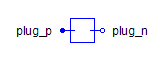Orifice with Pressure-Dependent Flow - MapleSim Help

Orifice with Pressure-Dependent Flow

Orifice with pressure-dependent flow rateDescription

The Orifice with Pressure-Dependent Flow (or Orifice Pressure Dependent Flow) component uses a user-supplied table to define the flow rate for a given pressure drop. It is a general two-port component and not restricted to be an orifice but is labeled as such and given an appropriate icon for the user's convenience. A similar part is also available as a check valve and a relief valve.

The flow rate through the component is computed as a function of the pressure difference between port A and port B from a data table containing values of pressure difference vs the corresponding flow rate.

If $q\left(-p\right)=-q\left(p\right)$, then the symmetric parameter can be assigned true and only the first quadrant of the $q$ vs $p$ curve needs to be defined in the table.Pressure vs Flow Rate For this component, the flow rate must be specified as a function of the pressure difference in the form of a table. The following parameters allows users to specify the necessary data for this calculation. Data Source: This parameter allows users to specify the nature of the source file for the Pressure vs. flow rate table. The available options are file, attachment, and inline. file: Use this option to specify a source file (an Excel or a CSV file) located on the hard drive. Use the $A\left(\mathrm{dP}\right)$ parameter to browse to and/or select the data file. The data file will not be attached to the MapleSim model. attachment: Use this option to specify a data file (an Excel or a CSV file) that is attached to the MapleSim model. To be used as an attachment, data files must be attached to the MapleSim model and be categorized under Data Sets. The attached files in a MapleSim model can be viewed in the Attachments pane under the Data Sets section. For more information, see Attaching a File to a Model. If the required file is not already attached to the MapleSim model, use the $A\left(\mathrm{dP}\right)$ parameter to attach a new file. Note: If the file option is used, subsequent changes to the source file will be incorporated into MapleSim simulations If the attachment option is used, subsequent changes to the source file will not affect the version attached to the MapleSim model. To ensure updates to the source files are incorporated in the simulation, the file must be reattached. The Data Generation App can be used to generate table data from mathematical functions using Maple code. For more information about MapleSim apps, see Opening MapleSim Apps and Templates. inline: Use this option to directly specify the pressure vs. area relationship in the form of a table. In the $A\left(\mathrm{dP}\right)$ parameter, enter an $m×2$  matrix. The first column must contain values of pressure difference and the second column must contain corresponding values of the area. Note: To change the dimensions of table, right-click (Control-click for Mac®) the parameter field and select Edit Matrix Dimensions. In the Matrix Dimensions dialog, enter values for the number or rows and columns and then click OK. Skip rows: This option allows users to specify the number of top rows to be ignored during the calculations. This option can be useful when the Excel file contains header information. Smooth: The smooth option specifies how the data table is interpolated. Pressure difference unit: This option specifies the unit of pressure used in the supplied data. Flow unit: This option specifies the unit of flow rate used in the supplied data.Optional Volumes The boolean parameters Use volume A and Use volume B, when true, add optional volumes ${V}_{A}$  and ${V}_{B}$ to ports A and B, respectively. See Port Volumes for details. If two orifices or valves are connected, enabling a volume at the common port reduces the stiffness of the system and improves the solvability.Equations $p={p}_{A}-{p}_{B}$ $q={q}_{A}-{q}_{\mathrm{VA}}=-{q}_{B}+{q}_{\mathrm{VB}}=\left(\left\{\begin{array}{cc}\mathrm{sign}\left(p\right)& \mathrm{symmetric}\\ 1& \mathrm{otherwise}\end{array}\right)\cdot \mathrm{Interpolate1D}\left(q\left(\mathrm{dP}\right),\left(\left\{\begin{array}{cc}\left|p\right|& \mathrm{symmetric}\\ p& \mathrm{otherwise}\end{array}\right)\cdot \mathrm{pressUnit},2,\mathrm{smoothness},\mathrm{skipRows}\right)$ ${q}_{\mathrm{VA}}=\left\{\begin{array}{cc}\frac{\mathrm{d}{V}_{{f}_{A}}}{\mathrm{d}t}& \\mathrm{seVolumeA}=\mathrm{true}\\ 0& \mathrm{otherwise}\end{array}\phantom{\rule[-0.0ex]{4.0ex}{0.0ex}}{q}_{\mathrm{VB}}=\left\{\begin{array}{cc}\frac{\mathrm{d}{V}_{{f}_{B}}}{\mathrm{d}t}& \\mathrm{seVolumeB}=\mathrm{true}\\ 0& \mathrm{otherwise}\end{array}$ ${V}_{{f}_{A}}=\left\{\begin{array}{cc}{V}_{A}\left(1+\frac{{p}_{A}}{\mathrm{El}}\right)& \\mathrm{seVolumeA}=\mathrm{true}\\ 0& \mathrm{otherwise}\end{array}\phantom{\rule[-0.0ex]{4.5ex}{0.0ex}}{V}_{{f}_{B}}=\left\{\begin{array}{cc}{V}_{B}\left(1+\frac{{p}_{B}}{\mathrm{El}}\right)& \\mathrm{seVolumeB}=\mathrm{true}\\ 0& \mathrm{otherwise}\end{array}$Variables

 Name Value Units Description Modelica ID $p$ $\mathrm{Pa}$ Pressure drop from A to B p $q$ $\frac{{m}^{3}}{s}$ Flow rate from port A to port B q ${q}_{\mathrm{summary}}$ $q\mathrm{flowScaleSummary}$ $\frac{{m}^{3}}{s}$ Internal flow sensor q_summary ${\mathrm{dp}}_{\mathrm{summary}}$ $p\mathrm{pressScaleSummary}$ $\mathrm{Pa}$ Internal pressure difference sensor dp_summaryConnections

 Name Description Modelica ID $\mathrm{portA}$ Upstream hydraulics port portA $\mathrm{portB}$ Downstream hydraulic port portBParametersFlow Rate Vs. Pressure

 Name Default Description Modelica ID $\mathrm{symmetric}$ $\mathrm{true}$ True (checked) the data for positive pressure difference used for both directions symmetric Data source inline Data source datasourcemode $q\left(\mathrm{dP}\right)$ $\left[100.,0.001\right]$ Data set table Skip rows $0$ Number of rows to skip skipRows Smooth $0$ Smoothness of table interpolation smoothness Pressure difference unit $\mathrm{kPa}$ Unit of pressure used in the data pressUnit $\mathrm{flowUnit}$ ${\mathrm{cm}}^{2}$ Unit flow rate used in the data flowUnitOptional Volumes

 Name Default Units Description Modelica ID $\mathrm{useVolumeA}$ $\mathrm{false}$ True (checked) means a hydraulic volume chamber is added to portA useVolumeA ${V}_{A}$ $1·{10}^{-6}$ ${m}^{3}$ Volume of chamber A Va $\mathrm{useVolumeB}$ $\mathrm{false}$ True (checked) means a hydraulic volume chamber is added to portB useVolumeB ${V}_{B}$ $1·{10}^{-6}$ ${m}^{3}$ Volume of chamber B Vb